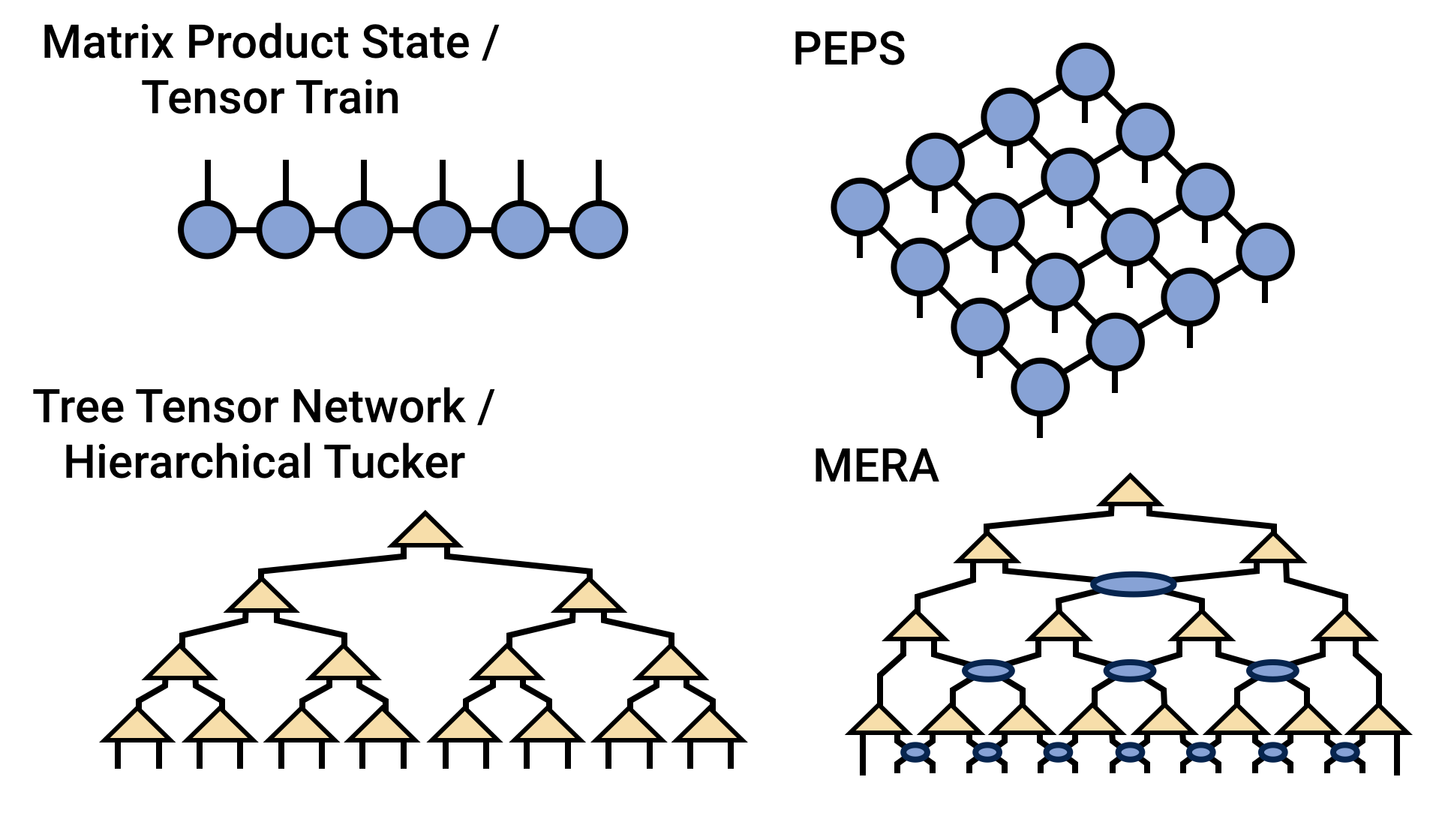About Contribute Source

### Welcome to the Tensor NetworkThis site is a resource for tensor network algorithms, theory, and software.

Tensor networks are factorizations of very large tensors into networks of smaller tensors, with applications in applied mathematics, chemistry, physics, machine learning, and many other fields.

Fundamentals:

Types of Tensor Networks:

Tensor Network Algorithms:

Computing with Tensor Networks:

Applications of Tensor Networks: